# SAT Math Multiple Choice Question 988: Answer and Explanation

### Test Information

Question: 988

8. Davis drew a unit circle and labeled the cosine and sine of 45° as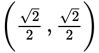. Assuming that Davis is correct, which of the following statements must be true?

• A.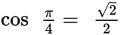• B.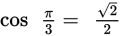• C.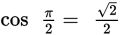• D.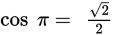Explanation:

A

Difficulty: Easy

Category: Additional Topics in Math / Trigonometry

Strategic Advice: Skim the answer choices to see what you're dealing with. All the values on the right-hand side of the equal sign are the same, so all you need to do is convert 45° to radians.

Getting to the Answer: Because π = 180°, converting between radians and degrees always involves using one of the two ratios,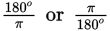. Use the ratio that will cancel the unit you want to get rid of (degrees):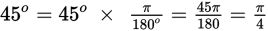. Therefore: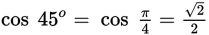, which is (A).

You may not have recognized that. If not, divide easy numbers (such as 5 or 9) out of the numerator and denominator until you reach one of the answer choices: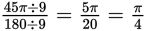.